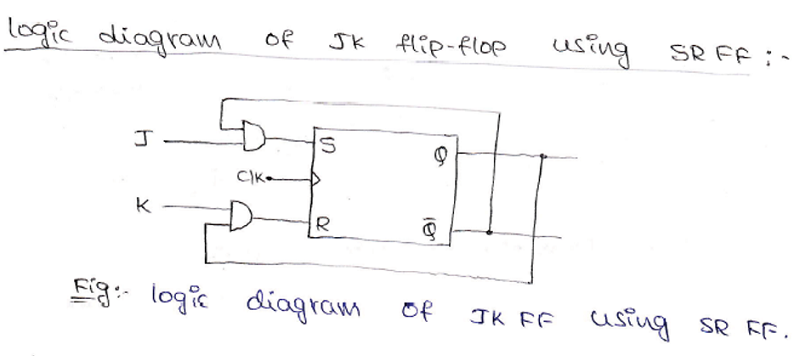# Conversion of Flip-Flops in Digital Electronics

In this tutorial, we will learn about the procedure of conversion flip-flops, and how one type of flip-flop can be converted into another type of flip flops in Digital Electronics. By Saurabh Gupta Last updated : May 11, 2023

## Flip-Flops Conversion Procedure

To convert one kind of flip-flop into another kind of flip-flop, we need to design a combinational circuit. The inputs of the required flip-flop are fed in as inputs to this combinational circuit, which are connected to the inputs of given flip-flop and the output of the combinational circuit is connected to the inputs of the given flip-flop, then the output of the given flip-flop will act as the output of the required flip-flop. A general schematic model for such conversion is depicted in the below-given figure: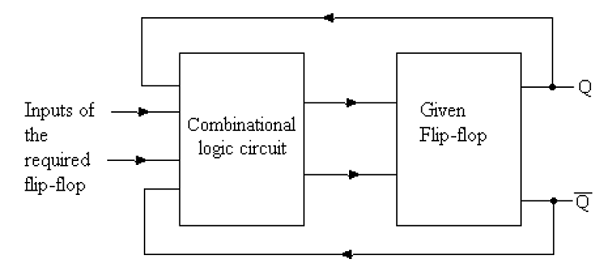To design the combinational circuit, we require a Boolean expression which is obtained using the excitation table of both given flip-flop and required flip-flop. The Boolean expressions are obtained by solving the K-Map drawn for the inputs and outputs of a given flip-flop.

## Excitation Table of flip-flops

Unlike the case of truth table, where we consider the combination of inputs given and the present state of the flip-flop to predict the output of the flip-flop.

In an excitation table, we take into account the present state and next state output, and then try to predict what inputs must be provided, so that we can get that particular next state output for a specific set of present state given.

### 1. Excitation Table of SR Flip-Flop

Excitation table for SR flip-flop can be drawn as: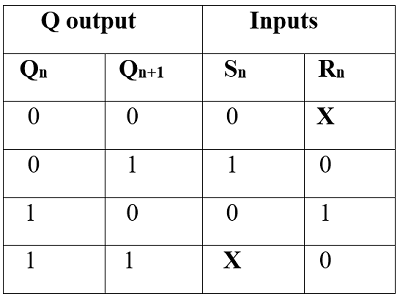### 2. Excitation Table of D (Delay) Flip-Flop

Excitation table for D flip-flop can be drawn as: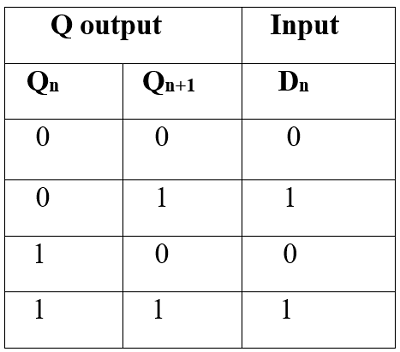### 3. Excitation Table of JK Flip-Flop

Excitation table for JK flip-flop can be drawn as: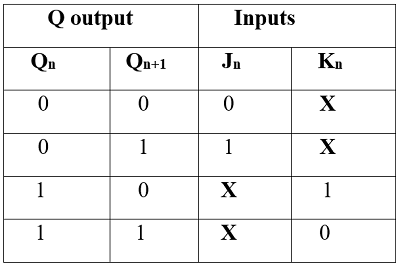### 4. Excitation Table of T (Toggle) Flip-Flop

Excitation table for T flip-flop can be drawn as: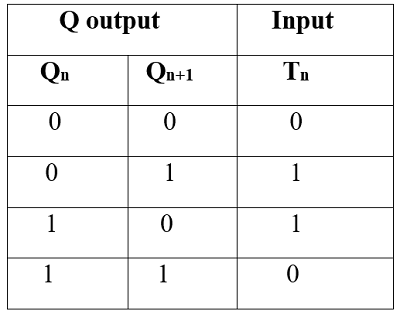## Convert One Flip-Flop to Another Flip-Flop

The following are the steps to convert one flip-flop to another flip-flop:

1. Make a table of the required flip-flop.
2. Using the excitation table of the given flip-flop write the corresponding outputs.
3. By drawing the K-Maps obtain the required Boolean expression for output.
4. Draw the combinational circuit using the obtained Boolean expression.

### Convert SR flip-flop to JK flip-flop

#### Solution

By following the above-given steps, conversion can be made as:

Step 1: Truth Table of required flip-flop (JK flip-flop) is given as: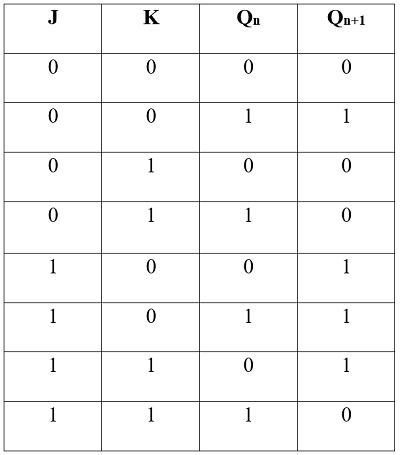Step 2: Using the excitation of given SR flip-flop, find the SR values, and complete the conversion table as: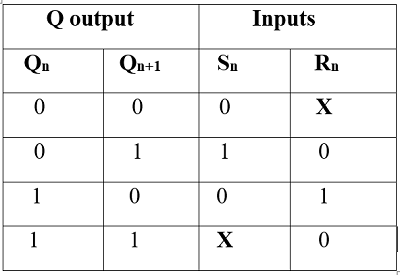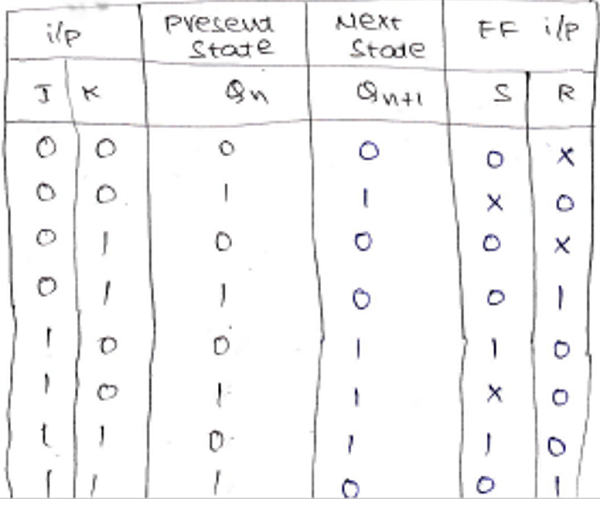Step 3: Solving K-Map for S and R values separately to get the Boolean expression.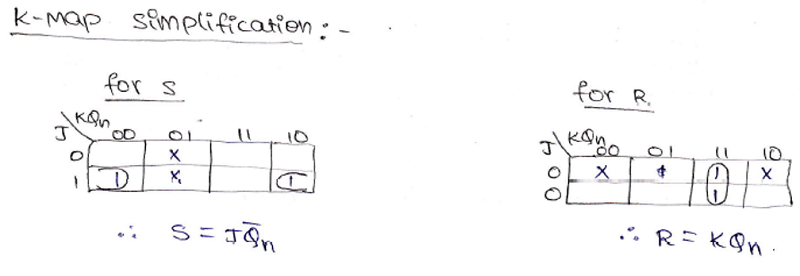Step 4: Making the logic circuit to implement the Combinational circuit using obtained Boolean expressions from K-Map.